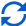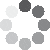Enter parameters Angle difference
Angle difference
Angle change:
deg
• degrees (deg)
• turns (tr)
• minutes of arc (arcmin)
• seconds of arc (arcsec)
Time:
sec
• seconds (sec)
• minutes (min)
• hours (hr)
• days (day)
CalculateResult
Angular Velocity:
0
• rotations per minute (rpm)
• degrees per second (deg/s)
• hertz (hz)
Linear Velocity:
m/s
• feet per second (ft/s)
• feet per minute (ft/m)
• feet per hour (ft/hr)
• miles per hour (mph)
• meters per second (m/s)
• meters per minute (m/m)
• kilometers per minute (km/m)
• kilometers per hour (km/h)
m
• inches (in)
• feet (ft)
• yards (yd)
• miles (mi)
• nautical miles (nmi)
• millimeters (mm)
• centimeters (cm)
• meters (m)
• kilometers (km)
CalculateResult
Angular Velocity:
0
• rotations per minute (rpm)
• degrees per second (deg/s)
• hertz (hz)(No Ratings Yet)Loading...
Real-time graphics. Make the calculations and see the changes.
Real-time graphics. Make the calculations and see the changes.
Embed this calculator on your site!Add live graphics
Copied to clipboard! PreviewWhat's wrong?
Minimum 5 characters!